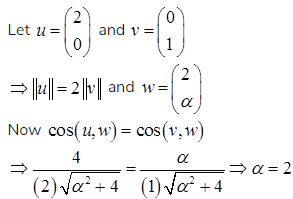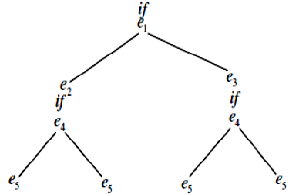# GATE Solved Paper 2017-19 - GATE 2017 Shift 1

>>>>>>>>GATE 2017 Shift 1

• A

1• B

2• C

3• D

4• Option : C
• Explanation : ((strlen(s) – strlen(t)) > c) ? strlen (s) : strlen (t)
= (3 – 5 > 0)
= (-2 > 0)
Important point here is while comparing -2 with c, result will be a positive number as c is unsigned. So, out of these two, strlen (s) will be printed. Therefore, option c is correct

• A

I only• B

II only• C

I and II• D

Neither I nor II• Option : A
• Explanation :
Union of context free language is also context free language.
L1 = { an bn cm| m >= 0 and n >= 0 } and
L2 = { am bncn| n >= 0 and m >= 0 }
L3 = L1 ∪ L2 = { anbncm∪ ambncn| n >= 0, m >= 0 } is also context free.
L1 says number of a’s should be equal to number of b’s and L2 says number of b’s should be equal to number of c’s. Their union says either of two conditions to be true. So it is also context free language.
Intersection of CFG may or may not be CFG.
L3 = L1 ∩ L2 = { anbncn| n >= 0 } need not be context free

• A

2• B• C

1• D

-• Option : A
• Explanation :• A

128• B

512• C

1024• D

2048• Option : C
• Explanation :
For 2 “if statements”, 22 = 4 control flow paths are possible:So for 10 “If statements”, control flow paths will be there.

• Option : A
• Explanation :
Given,
if TS(T2) <TS(T1) then
T1 is killed
else T2 waits.
- T1 holds a lock on the resource R
- T2 has requested a conflicting lock on the same resource R
According to algo, TS(T2) <TS(T1) then T1 is killed else T2 will wait. So in both cases neither deadlock will happen nor starvation.
Therefore, option A is correct
Related Quiz.
GATE 2017 Shift 1